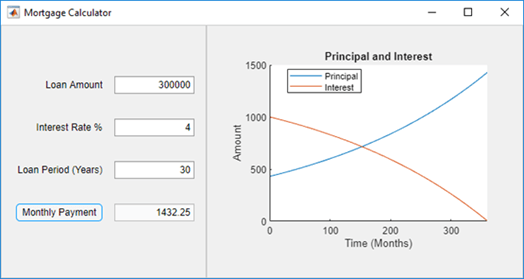# App That Calculates and Plots Data Based on Numerical Input

This app shows how to use numeric edit fields to create a simple mortgage amortization calculator. It includes the following components to collect user input, calculate monthly payments, and plot the principal and interest amounts over time:

• Numeric edit fields — allow users to enter values for the loan amount, interest rate, and loan period. MATLAB® automatically checks to make sure the values are numeric and within the range specified by the app. A fourth numeric edit field displays the resulting monthly payment amount based on the inputs.

• Push button — executes a callback function to calculate the monthly payment value.

• Axes — used to plot the principal and interest amounts versus mortgage installment.

To open the app in App Designer, enter this command in the MATLAB Command Window:

`openExample('matlab/MortgageCalculatorExample')`